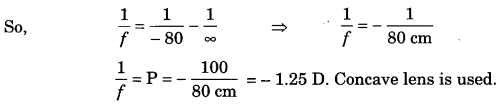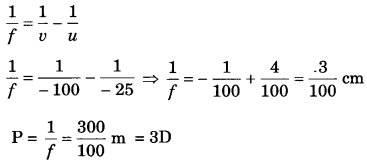# Bihar Board Class 10 Science Solutions Chapter 11 Human Eye and Colourful World

BSEB Bihar Board Class 10 Science Solutions Chapter 11 Human Eye and Colourful World Textbook Questions and Answers.

## Bihar Board Class 10 Science Solutions Chapter 11 Human Eye and Colourful World

### Bihar Board Class 10 Science Chapter 11 Human Eye and Colourful World InText Questions and Answers

In-text Questions (Page 190)

Question 1.
What is meant by power of accommodation of the eye?
The ability of the eye lens to adjust its focal length is called power of accommodation.

Question 2.
A person with a myopic eye cannot see objects beyond 1.2 m distinctly. What should be the type of the corrective lens used to restore proper vision?
Person should use concave lens.

Question 3.
What is the far point and near point of the human eye with normal vision?
Near point of normal human eye is 25 cm and far point is infinity.

Question 4.
A student has difficulty reading the blackboard while sitting in the last row. What could be the defect the child is suffering from? How can it be corrected?
The student is suffering from myopia. It can be corrected by using concave lens of suitable focal length.

### Bihar Board Class 10 Science Chapter 11 Human Eye and Colourful World Textbook Questions and Answers

Question 1.
The human eye can focus objects at different distances by adjusting the focal length of the eye lens. This is due to
(a) presbyopia
(b) accommodation
(c) near-sightedness
(d) far-sightedness
(b) accommodation

Question 2.
The human eye forms the image of an object at its
(a) cornea
(b) iris
(c) pupil
(d) retina

Question 3.
The least distance of distinct vision for a young adult with normal vision is about
(a) 25 m
(b) 2.5 cm
(c) 25 cm
(d) 2.5 m
(c) 25 cm

Question 4.
The change in focal length of an eye lens is caused by the action of the
(a) pupil
(b) retina
(c) ciliary muscles
(d) iris
(c) ciliary muscles

Question 5.
A person needs a lens of power -5.5 dioptres for correcting his distant vision. For correcting his near vision he needs a lens of power +1.5 dioptre. What is the focal length of the lens required for correcting (i) distant vision, and (ii) near vision?
(i) For distant vision P = -5.5D
f = $$\frac{100}{P}=\frac{100}{5.5}$$ = -18.2 cm

(ii) For near vision P = 1.5 D
f = $$\frac{100}{P}=\frac{100}{1.5}$$ = 66.7 cm

Question 6.
The far point of a myopic person is 80 cm in front of the eye. What is the nature and power of the lens required to correct the problem?
Distance of far point, x = 80 cm, P = ?
The lens will form image at 80 cm of the object lying at ∞
Here, υ = – 80 cm, u = ∞ cm and $$\frac{1}{f}=\frac{1}{v}-\frac{1}{u}$$Question 7.
Make a diagram to show how hypermetropia is corrected. The near point of a hypermetropic eye is 1 m. What is the power of the lens required to correct this defect? Assume that the near point of the normal eye is 25 cm.
For diagram Refer Q No. 1 (Long Answer Questions)
Distance of near point = 1 m = 100 cm
Here u = – 1 m = – 100 cm, υ = – 25 cmQuestion 8.
Why is a normal eye not able to see clearly the objects placed closer than 25 cm?
This is because the focal length of the eye lens cannot be decreased below a certain minimum limit i.e., 25 cm.

Question 9.
What happens to the image distance in the eye when we increase the distance of an object from the eye?
The image distance in the eye is fixed and equal to the distance of the retina from the eye lens. When the object distance increases focal length of the eye lens increases.

Question 10.
Why do stars twinkle?
The twinkling of a star is due to atmospheric refraction of starlight. The starlight, on entering the earth’s atmosphere undergoes refraction continuously before it reaches the earth. The atmospheric refraction occurs in a medium of gradually changing refractive index. Since the atmosphere bends starlight towards the normal, the apparent position of the star is slightly different from its actual position. Since the physical conditions of the earth’s atmosphere are not stationary. Apparent position changes and star appears twinkling.

Question 11.
Explain why the planets do not twinkle.
The planets are much closer to the earth, and are thus seen as extended sources. If we consider a planet as a collection of a large number of point-sized sources of light, the total variation in the amount of light entering our eye from all the individual point-sized sources will average out to zero, thereby nullifying the twinkling effect.

Question 12.
Why does the Sun appear reddish early in the morning?
In the morning, the Sun lies near the horizon, most of the blue light and shorter wavelengths are scattered away by the particles. Therefore, the light that reaches our eyes is of longer wavelength. This gives rise to the reddish appearance of the Sun.

Question 13.
Why does the sky appear dark instead of blue to an astronaut?